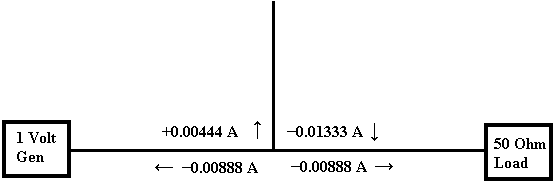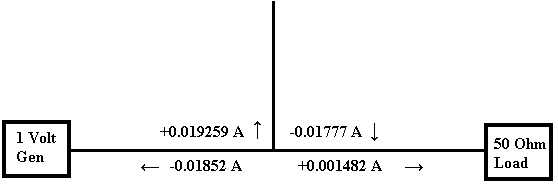# How does a stub filter work page 2

State at 360 degrees

5) When the signal at the junction has reached 360 degrees, the incident current will again be at the positive peak and the currents due to the incident wave will be as per Figure 8.

Figure 8The current at 180 degrees in Figure 5 will now have travelled to the end of the stub filter and back. This will give currents at the T junction as per Figure 9.

Figure 9The current at zero degrees in Figure 3 will now have travelled to the end of the stub filter and back two times, a total of 360 degrees. This will give currents as per Figure 10 at the T junction.

Figure 10The resulting sum current of these three signals at 360 degrees will be as per Figure 11. It can be seen that the current reflected back to the 1 Volt generator is increasing toward the generator input current and the signal going to the 50 Ohm load is tending towards zero. Whilst the current in the stub filter is also increasing towards the generator current.

Figure 11The voltages are now calculated from the currents and shown in Figure 12. This is to give reference values for the following measurement paragraphs.

Figure 121  2  3  4  5  >  Page

Technical Article Index